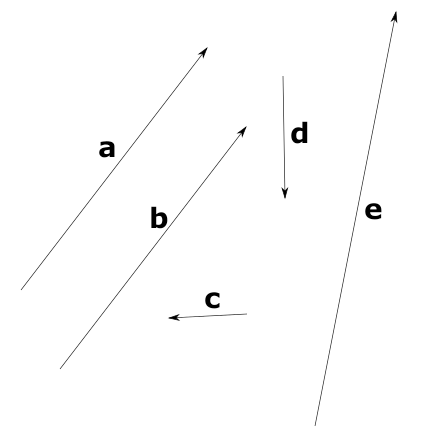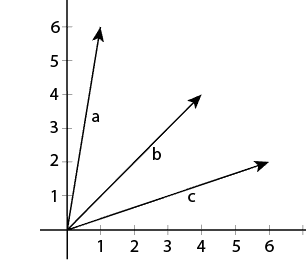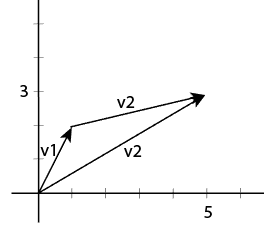eng   рус

# Vectors in Computer Science

Previous tutorial: Greek alphabet

Vector is an object that has direction and length (magnitude). We can represent points in space as vectors and additionally we can use vectors to represent physics forces.

Also in this tutorial we'll discuss the implementation of vector class in Python.

In printed text for vectors we use Latin letters in bold: a, b, v... In handwriting vectors are represented as: a or a.

On graphs vectors are drawn like line segments with arrow. Here how it looks like:As you can see there is no coordinate system as vectors represent direction and magnitude and not position. Though we can represent vectors in space. Vectors have components that can be interpreted as coordinates. Here is example of 2-dimensional (on plane) vector: a = (1, 1), and here - 3-dimensional (in 3d space) vector: b = (3, 2, 1). These numbers are correspond to coordinates of the point that is located at the end tip of vector if we put beginning of the vector in the origin of coordinate space. Let's see examples:Here we have vectors: a = (1,6), b = (4,4), c = (6,2).

Such vectors are called radius vectors and they are bridge between just magnitude/direction to points. With radius vectors we gain access to very powerful tools in 2d/3d graphics.

## Operations on vectors

We can do many things with vectors: find it's length (magnitude), sum (to find new position on plane or in space), multiply. Though the applications for these operations we'll find in next tutorials, after we'll discuss matrices and we'll be able to transform (change position, rotate) vectors.

### Magnitude of the vector

Magnitude (length of vector) is denoted as ||v||. We can calulate it by using Pythagoras' theorem:

$$\lVert v \rVert = \sqrt{v_x^2 + v_y^2}$$

We can sum vectors. The result is the new vector which components are the sum of appropriate components of initial vectors.

v1 = (1,2) v2 = (4,1) v3 = v1 + v2 = (v1x + v2x, v1y + v2y) = (1+4, 2+1) = (5, 3)

On the graph sum can be represented like this: we move the beginning of the second vector to the end of the first vector. Sum vector starts at the beginning of the first vector and ends at the end of the second vector. It will look like this:### Vector subtraction

Vector subtraction is just a case of the sum:

v1 - v2 = v1 + (-v2) v1 = (5,3) v2 = (1,1) v3 = v1 - v2 = (v1x - v2x, v1y - v2y) = (5-1, 3-1) = (4, 2)

### Dot product

Vectors have two multiplication operations. The result of one is scalar (simple number) - it's called dot product. Another one is cross product - the result of it is anothe vector. Let's start with dot product. We can calculate dot product in two ways:

$$a \cdot b = \lVert a \rVert \lVert b \rVert \cos \theta$$ $$a \cdot b = a_x b_x + a_y b_y$$

### Cross product

The result of cross product is anothe vector that's perpendicular to both initial:

$$a \times b = \lVert a \rVert \lVert b \rVert \sin(\theta) n$$

Also we can calculate cross product this way:

$$c_x = a_y b_z − a_z b_y$$ $$c_y = a_z b_x − a_x b_z$$ $$c_z = a_x b_y − a_y b_x$$

The order in which we make cross product matters. If we swap vectors, the result vector will have opposite direction

### Normalizing vectors

Normalized vector - is the vector that points to the same difection but has unit length. To find normalized vector we need to divide each component of vector by vector's magnitude.

## Conclusion

We'll use vectors a lot in following tutorials. They are essential part in graphics programming.